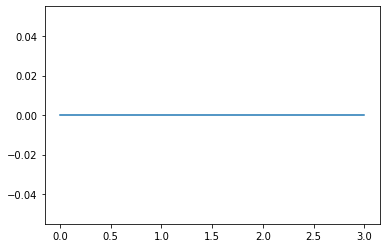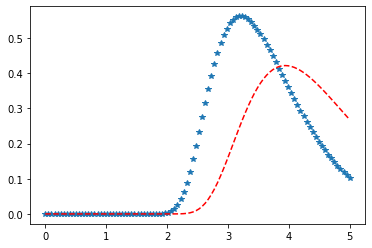# Python – Kolmogorov-Smirnov Distribution in Statistics

scipy.stats.kstwobign() is Kolmogorov-Smirnov two-sided test for large N test that is defined with a standard format and some shape parameters to complete its specification. It is a statistical test that measures the maximum absolute distance of the theoretical CDF from the empirical CDF.

Parameters :

q : lower and upper tail probability
x : quantiles
loc : [optional]location parameter. Default = 0
scale : [optional]scale parameter. Default = 1
size : [tuple of ints, optional] shape or random variates.

Results : kstwobign continuous random variable

Code #1 : Creating kstwobign continuous random variable

 `# importing library ` ` `  `from` `scipy.stats ``import` `kstwobign   ` `   `  `numargs ``=` `kstwobign.numargs  ` `a, b ``=` `4.32``, ``3.18` `rv ``=` `kstwobign(a, b)  ` `   `  `print` `(``"RV : \n"``, rv)   ` ` `

Output :

```RV :
scipy.stats._distn_infrastructure.rv_frozen object at 0x000002A9D54959C8
```

Code #2 : kstwobign continuous variates and probability distribution

 `import` `numpy as np  ` `quantile ``=` `np.arange (``0.01``, ``1``, ``0.1``)  ` ` `  `# Random Variates  ` `R ``=` `kstwobign.rvs(a, b, scale ``=` `2``, size ``=` `10``)  ` `print` `(``"Random Variates : \n"``, R)  `

Output :

```Random Variates :
[3.88510141 3.48394857 3.66124797 3.88484201 3.86533511 3.21176073
4.10238585 3.42397866 3.85111721 4.36433596]
```

Code #3 : Graphical Representation.

 `import` `numpy as np  ` `import` `matplotlib.pyplot as plt  ` `    `  `distribution ``=` `np.linspace(``0``, np.minimum(rv.dist.b, ``3``))  ` `print``(``"Distribution : \n"``, distribution)  ` `    `  `plot ``=` `plt.plot(distribution, rv.pdf(distribution))  `

Output :

```Distribution :
[0.         0.06122449 0.12244898 0.18367347 0.24489796 0.30612245
0.36734694 0.42857143 0.48979592 0.55102041 0.6122449  0.67346939
0.73469388 0.79591837 0.85714286 0.91836735 0.97959184 1.04081633
1.10204082 1.16326531 1.2244898  1.28571429 1.34693878 1.40816327
1.46938776 1.53061224 1.59183673 1.65306122 1.71428571 1.7755102
1.83673469 1.89795918 1.95918367 2.02040816 2.08163265 2.14285714
2.20408163 2.26530612 2.32653061 2.3877551  2.44897959 2.51020408
2.57142857 2.63265306 2.69387755 2.75510204 2.81632653 2.87755102
2.93877551 3.        ]
```Code #4 : Varying Positional Arguments

 `import` `matplotlib.pyplot as plt  ` `import` `numpy as np  ` `    `  `x ``=` `np.linspace(``0``, ``5``, ``100``)  ` `    `  `# Varying positional arguments  ` `y1 ``=` `kstwobign .pdf(x, ``1``, ``3``)  ` `y2 ``=` `kstwobign .pdf(x, ``1``, ``4``)  ` `plt.plot(x, y1, ``"*"``, x, y2, ``"r--"``)  `

Output :Attention geek! Strengthen your foundations with the Python Programming Foundation Course and learn the basics.

To begin with, your interview preparations Enhance your Data Structures concepts with the Python DS Course.

My Personal Notes arrow_drop_upCheck out this Author's contributed articles.

If you like GeeksforGeeks and would like to contribute, you can also write an article using contribute.geeksforgeeks.org or mail your article to contribute@geeksforgeeks.org. See your article appearing on the GeeksforGeeks main page and help other Geeks.

Please Improve this article if you find anything incorrect by clicking on the "Improve Article" button below.

Article Tags :

Be the First to upvote.

Please write to us at contribute@geeksforgeeks.org to report any issue with the above content.